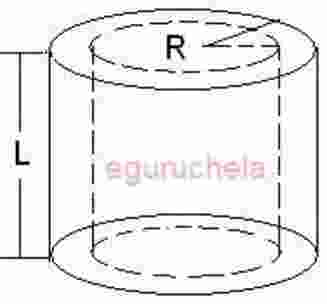# Calculate Hollow cylinder volume, area, surface area and circumference

Calculate the various properties of a tube, also called a pipe or hollow cylinder for given values

 Cylinder outer radius value (r1): m Cylinder inner radius value (r2): m Cylinder height (L): m

 Circumference outer (C1) m Circumference inner (C2) m Lateral Surface Area outer (L1) m2 Lateral Surface Area inner (L2) m2 Area (A) m2 Volume (V) m3 Wall thickness of the tube m

This calculator will for inner-outer radius and height. The hollow circular cylinder is a three-dimensional solid confined by two parallel cylindrical surfaces having two parallel circular bases.

The shortest distance from the center of the circular base to the inner cylinder is called as inner radius, and the shortest distance from the center of the circular base to the outer cylinder is called outer radius.## The formulas and definitions

Area of a Hollow Cylinder:

2π ( r1 + r2)( r1 – r2 +h)

where $$\mathbf{r_{1}}$$ is the outer radius of the given cylinder, $$\mathbf{r_{2}}$$ is inner radius and $$\mathbf{h}$$ is height. $$\mathbf{C_{1}}$$ is the outer circumference and $$\mathbf{C_{2}}$$ is the inner circumference. L1 and L2 is the outer and inner surface areas respectively. t be the thickness of the cylinder ($$\mathbf{r_{1}- r_{2}}$$)

Area of Hollow Cylinder formulas:

The Circumference of a circle (C) is given by:

$$C = 2\pi r$$, therefore,$$C_{1} = 2\pi r_{1}$$ $$C_{2} = 2\pi r_{2}$$

The Lateral Surface Area (L),for a cylinder is:

$$L = C \times h = 2 \pi r h$$, therefore,
$$L_{1} = 2 \pi r_{1} h$$, the external curved surface area
$$L_{2} = 2 \pi r_{2} h$$, the internal curved surface area

Lateral Surface Area of a hollow cylinder = $$L = 2 \pi r_{1} h + 2 \pi r_{2} h$$

The Cross sectional Area:

Let A is the area of a cross-section of a hollow cylinder, $$\pi r^{2}$$, for a circle, therefore;
A1 = $$\pi r_{1}^{2}$$ for the area enclosed by $$r_{1}$$
A2 = $$\pi r_{2}^{2}$$for the area enclosed by $$r_{2}$$
A = A1 – A2 for the cross sectional area of hollow cylinder
A = $$\pi r_{1}^{2}- \pi r_{2}^{2} = \pi (r_{1}^{2}- r_{2}^{2})$$

Total Surface Area of a Hollow Cylinder:

=$$2 \pi h (r_{1}+ r_{2}) + 2 \pi (r_{1}^{2} – r_{2}^{2})$$
=$$2 \pi h (r_{1}+ r_{2}) + 2 \pi (r_{1} + r_{2}) (r_{1} – r_{2})$$
=$$2 \pi (r_{1}+ r_{2}) (h + r_{1} – r_{2})$$